Ministério da Ciência, Tecnologia, Inovações e Comunicações CBPFIndex CENTRO BRASILEIRO DE PESQUISAS FÍSICAS11/12/2023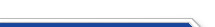Usuário: Senha: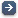Crie sua conta aqui · Busca rápida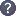Procurar por: Publicações Projetos EventosIr para a busca avançada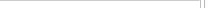Notas de Física
 Beyond the $10$-fold way: $13$ associative ${\mathbb Z}_2\times{\mathbb Z}_2$-graded superdivision algebras Data do cadastro: 08/12/2021 Publicação/Divulgação: 08/12/2021 Resp. pelo cadastro: Status atual: Publicado Ano da publicação: 2021 Descrição: The $10$-fold way" refers to the combined classification of the $3$ associative division algebras (of real, complex and quaternionic numbers) and of the $7$, ${\mathbb Z}_2$-graded, superdivision algebras (in a superdivision algebra each homogeneous element is invertible). \\ The connection of the $10$-fold way with the periodic table of topological insulators and superconductors is well known. Motivated by the recent interest in ${\mathbb Z}_2\times{\mathbb Z}_2$-graded physics (classical and quantum invariant models, parastatistics) we classify the associative ${\mathbb Z}_2\times {\mathbb Z}_2$-graded superdivision algebras and show that $13$ inequivalent cases have to be added to the $10$-fold way. Our scheme is based on the alphabetic presentation of Clifford algebras", here extended to graded superdivision algebras. The generators are expressed as equal-length words in a $4$-letter alphabet (the letters encode a basis of invertible $2\times 2$ real matrices and in each word the symbol of tensor product is skipped). The $13$ inequivalent \zzg superdivision algebras are split into real series ($4$ subcases with $4$ generators each), complex series ($5$ subcases with $8$ generators) and quaternionic series ($4$ subcases with $16$ generators). Número: CBPF-NF-004/21 Autores: Z. Kuznetsova; F. Toppan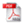Download do PDF (1.419 KB)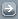Todos EventosTodos PublicaçõesTodasArtigos de Div. CientíficaArtigos em Revistas Nac.Artigos em Revistas Int.Artigos em Eventos Nac.Artigos em Eventos Int.Livros InteirosCapítulos de LivroPropriedades IntelectuaisÁudioPatentesProgramas de computadorModelos de utilidadeMarcasOutras prop. intel.TecnologiasKnow-howServiços tecnológicosTecnologias inovadorasTeses de DoutoradoDissertações de MestradoProjetos de GraduaçãoCiência e SociedadeDocumentos HistóricosMonografiasNotas de FísicaNotas TécnicasOutras Produções
 · Principal    |    CBPF    |    · Copyright 2004 - CBPF. Rio de Janeiro/BrasilCentro Brasileiro de Pesquisas Físicasv 1.1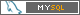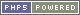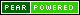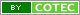Pï¿½g. gerada em 0.01 s Total de consultas SQL: 23